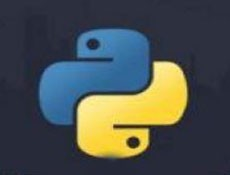# python可以继承父类方法吗• silencement   2019-08-24 15:19:51361python继承,调用父类属性方法

```class Animal:
def heshui(self):
print('动物正在喝水')

class Cat(Animal):
pass```

```#接上面代码

cat = Cat()

cat.heshui()

>>>动物正在喝水```

```class Animal:
def heshui(self):
print('动物正在喝水')

class Cat(Animal):
def heshui(self):
print('猫正在喝水')
cat = Cat()
cat.heshui()

>>>猫正在喝水```

```class Animal:
def heshui(self):
print('动物正在喝水')

class Cat(Animal):
def heshui(self):
super().heshui()
cat = Cat()
cat.heshui()

>>>动物正在喝水```

```class Animal:
def __heshui(self):
print('动物正在喝水')class Cat(Animal):
def heshui(self):
super()._Animal__heshui()

cat = Cat()
cat.heshui()>>>动物正在喝水```

```class Animal:
def __init__(self):
self.a = 'aaa'

class Cat(Animal):
def __init__(self):
pass

cat = Cat()
print(cat.a)

>>>AttributeError: 'Cat' object has no attribute 'a'```

```class Animal:
def __init__(self):
self.a = 'aaa'class Cat(Animal):
def __init__(self):
super().__init__()  #也可以用 Animal.__init__(self)  这里面的self一定要加上

cat = Cat()
print(cat.a)>>>aaa```